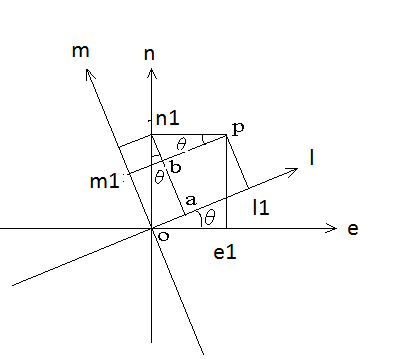基于WGS-84坐标系的坐标转换解决方案

1、方案背景2、算法原理l1=oa+al1;

m1=an1-bn1;

oa=n1*sin(θ) , al1=e1*cos(θ)

an1=n1*cos(θ), bn1= e1*sin(θ)

l1 = oa + al1 = n1*sin(θ)+e1*cos(θ)

m1 = an1-bn1 = n1*cos(θ)-e1*sin(θ)

2、实施过程